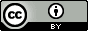## Tuesday, December 9, 2014

### QM computed standard free energy changes and pH

2015.01.25:  I have summarized discussion of this and related issues in this paper.  I have also made some changes in the post to reflect what I have learned since I wrote it.

The problem
Thermodynamics involving ionizable functional group at constant pH require special considerations. Robert Alberty has written extensively on this topic (example) but I confess I had some trouble relating the work to quantum chemical calculations.  This post aims to do just that.

Let's say you want to compute the standard free energy change at pH 7 for this equilibrium

$\mathrm{B \rightleftharpoons HA}$

where molecule $\mathrm{B}$ can be converted to an acid $\mathrm{HA}$, which is in equilibrium with it conjugate base and thus pH-dependent.

$\mathrm{HA \rightleftharpoons A^- + H^+}$

The apparent equilibrium constant ($K^\prime$) is

$K^\prime=\mathrm{\frac{[HA]+[A^-]}{[B]}}$

How to compute the corresponding standard free energy change?:

$\Delta G^{\prime\circ}=-RT\ln(K^\prime)$

The Solution
$K^\prime$ can be rewritten as

$K^\prime=K+\frac{KK_a}{[\mathrm{H^+}]}$

where

$K=\mathrm{\frac{[HA]}{[B]}}=\exp{(-\Delta G^\circ/RT)}$

and

$K_a=\mathrm{\frac{[A^-][H^+]}{[HA]}}=\exp{(-\Delta G^\circ_a/RT)}$

Thus,

$K^\prime=\exp{(-\Delta G^\circ/RT)} + \exp{(-\Delta G^\circ/RT)}\exp{(-\Delta G^\circ_a/RT)}10^{\mathrm{pH}}$

$=\exp{(-\Delta G^\circ/RT)} + \exp{(-(\Delta G^\circ+\Delta G^\circ_a-RT\ln(10)\mathrm{pH})/RT)}$

$=\frac{\exp{(-G^\circ(\mathrm{HA})/RT)}+\exp{(-G^{\prime\circ}(\mathrm{A^-})/RT)} }{\exp{(-G^\circ(\mathrm{B})/RT)}}$

where

$G^{\prime\circ}(\mathrm{A^-})=G^{\circ}(\mathrm{A^-})+[G^{\circ}(\mathrm{H^+})-RT\ln(10)\mathrm{pH}]$  (1)

Thus

$\Delta G^{\prime\circ}= G^{\prime\circ}(\mathrm{\overline{HA}})-G^\circ(\mathrm{B})$ (2)

where

$G^{\prime\circ}(\mathrm{\overline{HA}})=-RT\ln \left( \exp{(-G^\circ(\mathrm{HA})/RT)}+\exp{(-G^{\prime\circ}(\mathrm{A^-})/RT)} \right)$ (3)

Here $\mathrm{\overline{HA}}$ refers to "$\text{HA and A}$"

An example
How do you compute the standard free energy change in aqueous solution at pH 7 using QM for this reaction?:

$\mathrm{\text{HC(=O)-}NH_2+ H_2O \rightleftharpoons HCOOH + NH_3}$

First you optimize each molecule and perform the vibrational analysis to get the free energies.  You can account for solvation effects using a method like PCM or COSMO.  $\mathrm{HCOOH}$ and $\mathrm{NH_3}$ are acids and basis, respectively so you will also need the energies for $\mathrm{HCOO^-}$ and $\mathrm{NH_4^+}$

Most QM programs assume the molecules are in the gas phase when computing the Gibbs free energies so the standard state is 1 bar.  The free energies must be corrected for the solution standard state of 1 M:

$G^\circ(\mathrm{X}) = G^{\circ,\mathrm{1 bar}}(\mathrm{X})-RT\ln (V^{-1})$

where $V$ is the molar volume of an ideal at gas at your chosen temperature.  The exception is water since that is also the solvent.  Here the standard state is 55.34 M at 25C.

$G^\circ(\mathrm{H_2O}) = G^{\circ,\mathrm{1 bar}}(\mathrm{H_2O})-RT\ln ((55.34V)^{-1})$

$G^{\prime\circ}(\mathrm{HCOO^-})$ and is then computed using Eq (1). $G^{\circ}(\mathrm{H^+})$ is usually taken from the literature though estimates vary between -265.8 and -268.6 kcal/mol.

$G^{\prime\circ}(\mathrm{\overline{HCOOH}})$ can then be computed using Eq (3).  The electronic energy component of $G^{\circ}(\mathrm{X})$ can be quite large in magnitude and give some numerical problems when computing the exponential function so Eq (3) and be rewritten as

$G^{\prime\circ}(\mathrm{\overline{HA}})= G^\circ(\mathrm{HA})-RT\ln \left(1+\exp{(-(G^{\prime\circ}(\mathrm{A^-})-G^\circ(\mathrm{HA}))/RT)} \right)$

Following a derivation similar to the one at the beginning of this post,

$G^{\prime\circ}(\mathrm{NH_4^+})=G^{\circ}(\mathrm{NH_4^+})-[G^{\circ}(\mathrm{H^+})-RT\ln(10)\mathrm{pH}]$

and $G^{\prime\circ}(\mathrm{\overline{NH_3}})$ is computed by Eq (3)

Finally, we put everything together

$\Delta G^{\prime\circ}=G^{\prime\circ}(\mathrm{\overline{HCOOH}})+G^{\prime\circ}(\mathrm{\overline{NH_3}})- G^\circ(\mathrm{\text{HC(=O)-}NH_2})-G^\circ(\mathrm{H_2O})$

Another way
If you happen to know the pKa values (e.g. from experiment) of the ionizable species you can use this expression

$\Delta G^{\prime\circ}=\Delta A^{\circ}-RT\ln \left( 1+10^{\mathrm{pH}- pK_{a,\mathrm{HCOOH}}} \right)-RT\ln \left( 1+10^{ pK_{a,\mathrm{NH_4^+}}-\mathrm{pH}} \right)$

where

$\Delta G^{\circ}=G^{\circ}(\mathrm{HCOOH})+G^{\circ}(\mathrm{NH_3})- G^\circ(\mathrm{\text{HC(=O)-}NH_2})-G^\circ(\mathrm{H_2O})$